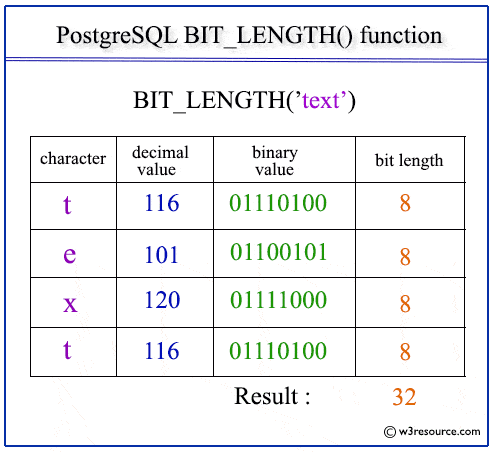# PostgreSQL BIT_LENGTH() function

## BIT_LENGTH() function

The PostgreSQL bit_length function is used to count the number of bits in a specified string.

Syntax:

``` bit_length (str1)
```

Parameters:

Name Description
str1 A string whose BIT_LENGTH value is to be retrieved.

PostgreSQL Version: 9.3

Pictorial Presentation of PostgreSQL BIT_LENGTH() functionExample: PostgreSQL BIT_LENGTH() function

The following PostgreSQL statement will return the length of the given string 'w3resource'' in bits, i.e. 80.

Code:

``````SELECT bit_length('w3resource') AS "bit_length";
```
```

Sample Output:

``` bit_length
------------
80
(1 row)
```
﻿